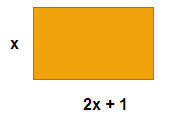# NCERT Solutions for Class 10 Maths Chapter 4 Exercise 4.1

In this page we have NCERT Solutions for Class 10th Maths: Chapter 4 - Quadratic Equations for EXERCISE 4.1 on page number 73 and 74. Hope you like them and do not forget to like , social_share and comment at the end of the page.

Question 1
Check whether the following are quadratic equations
1. (x+1)2=2(x-3)
2. x2 -2x=(-2)(3-x)
3. (x-2)(x+1)=(x-1)(x+3)
4. (x - 3)(2x +1) = x(x + 5)
5. (2x - 1)(x - 3) = (x + 5)(x - 1)
6. x2 + 3x + 1 = (x - 2)2
7. (x + 2)3 = 2x (x2 - 1)
8. x3 - 4x2 - x + 1 = (x - 2)3
Solution 1
we know that
ax2 +bx+c =0     where a≠0
1. (x+1)2=2(x-3) We know that
(a+b)2=a2+b2+2ab
⇒x2 + 2x+1=2x-6
Simplifying it
⇒;x2 +7=0
Since it is of a quadratic form : ax2 +bx+c   =0     where a≠0
with b=0
So it is a quadratic equation
2.  x2 -2x=(-2)(3-x)
Simplifying it
x2 -2x=-6+2x
⇒x2 -4x+6=0
Since it is a quadratic form
ax2 +bx+c   =0     where a≠0
So it is a quadratic equation
3.  x2 -2x=(-2)(3-x)
(x-2)(x+1)=(x-1)(x+3)
Multiplying both the factors
⇒x2 -2x+2+x= x2 +3x-x-3
Simplifying
-3x+1=0
It is not of the quadratic form
ax2 +bx+c   =0     where a≠0
So it is not a quadratic equation
4. (x - 3)(2x +1) = x(x + 5)
Multiplying both the factors
2x2+x-6x-3=x2+5x
Simplifying
x2 -10x-3=0
Since it is a quadratic form
ax2 +bx+c   =0     where a≠0
⇒So, it is a quadratic equation
5. (2x - 1)(x - 3) = (x + 5)(x - 1)
Multiplying both the factors on both sides
2x2 -6x-x+3=x2 -x+5x-5
⇒x2 -11x +8=0
Since it is a quadratic form
ax2 +bx+c   =0     where a≠0
So, it is a quadratic equation
6. x2 + 3x + 1 = (x - 2)2
We know that
(a+b)2=a2+b2+2ab
⇒x2 + 3x + 1 =x2-4x+4
⇒7x-3=0
Since it is not of quadratic form
ax2 +bx+c   =0     where a≠0
So, it is a not quadratic equation
7. (x + 2)3 = 2x (x2 - 1)
Important formula you must have remembered in old classes
(a+b)3= a3 +b3+3ab2+3a2b
⇒x3 +8+6x2+12x=2x3 -2x
Simplifying
x3-6x2 -14x-8=0
Since it is not of quadratic form
ax2 +bx+c   =0     where a≠0
So, it is a not quadratic equation
8. x3 - 4x2 - x + 1 = (x - 2)3
Important formula  you must have remembered in  old classes
(a-b)3= a3 -b3+3ab2-3a2b
x3 - 4x2 - x + 1 =x3-8-6x2+12x
Simplifying
2x2 -13x+9=0
Since it is a quadratic form
ax2 +bx+c   =0     where a≠0
So, it is a quadratic equation

Question 2
Represent the following situations in the form of quadratic equations :
1. The area of a rectangular plot is 528 m2. The length of the plot (in meters) is one more than twice its breadth. We need to find the length and breadth of the plot.
2. The product of two consecutive positive integers is 306. We need to find the Integers.
3. Rohan’s mother is 26 years older than him. The product of their ages (in years) 3 years from now will be 360. We would like to find Rohan’s present age.
4. A train travels a distance of 480 km at a uniform speed. If the speed had been 8 km/h less, then it would have taken 3 hours more to cover the same distance. We need to find the speed of the train
Solution
1.Let the breath of the plot= x  m
As per given condition in the question
Length  =2x+1
Now we know that Area is given by
A=LB
A=528 m2
So
528=(2x+1)x
⇒ x2+2x-528=0
2. let the two consecutive positive integers are x and x+1
The product of these would be
x(x+1)
It is given that product is 306
So
⇒ x(x+1)=306
⇒ x2+x-306=0
⇒ Which is a quadratic equation
3. Let Rohan present age=x year
Then Rohan Mother present age would =x+26
After 3 year,
Rohan age would be =x+3
Rohan mother’s age would be =x+26+3=x+29
According to question, The product of their ages (in years) =360
Then
(x+3)(x+29)= 360
Simplifying
⇒ x2 +29x +3x+87=360
⇒ x2 +32x -273=0
4. Let the speed of the train is x km/hr
Now distance travelled by the train=480 km
Few important formula here
Speed=Distance/time
Or Time= Distance /Speed
Case I
Time taken to travel 480 km by train will be =480/x
Case II
Now the speed of the train is reduced by 8 km/hr,
So speed would (x-8)
Now Time taken to travel 480 km will be =480/x-8
Now as per the question
480/(x-8) -  480/x  =3
⇒ [480x-480(x-8)]/x(x-8)  =3
⇒ 480x-480x+3840=3x(x-8)
⇒ 3x2 -24x-3840=0
⇒ Which is a quadratic equation

## Summary

1. NCERT Solutions for Class 10th Maths: Chapter 4 - Quadratic Equations Ex 4.1 has been prepared by Expert with utmost care. If you find any mistake.Please do provide feedback on mail.You can download this as pdf
2. This chapter 4 has total 4 Exercise 4.1 ,4.2,4.3 and 4.4. This is the First exercise in the chapter.You can explore previous exercise of this chapter by clicking the link belowGo back to Class 10 Main Page using below links

### Practice Question

Question 1 What is $1 - \sqrt {3}$ ?
A) Non terminating repeating
B) Non terminating non repeating
C) Terminating
D) None of the above
Question 2 The volume of the largest right circular cone that can be cut out from a cube of edge 4.2 cm is?
A) 19.4 cm3
B) 12 cm3
C) 78.6 cm3
D) 58.2 cm3
Question 3 The sum of the first three terms of an AP is 33. If the product of the first and the third term exceeds the second term by 29, the AP is ?
A) 2 ,21,11
B) 1,10,19
C) -1 ,8,17
D) 2 ,11,20